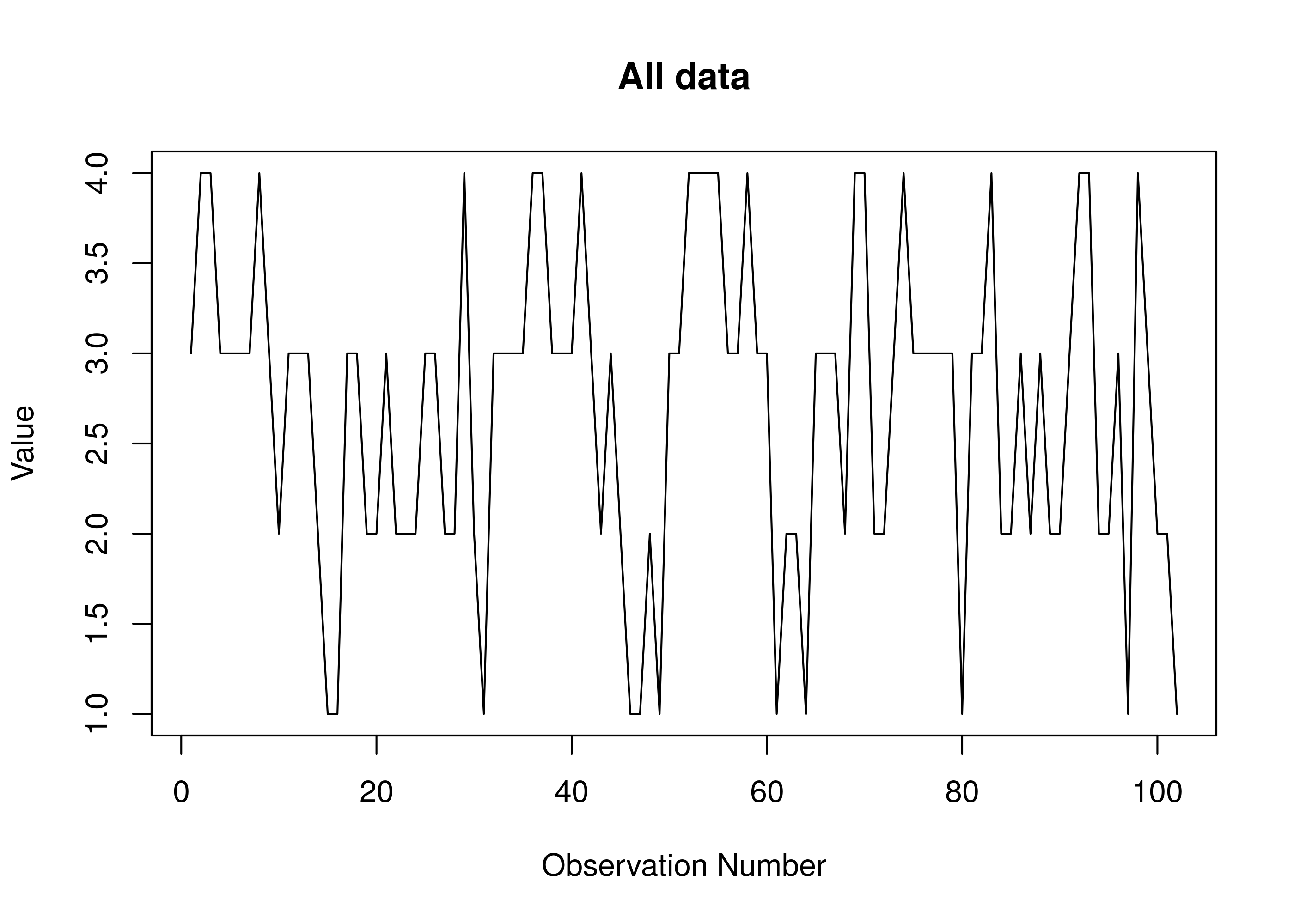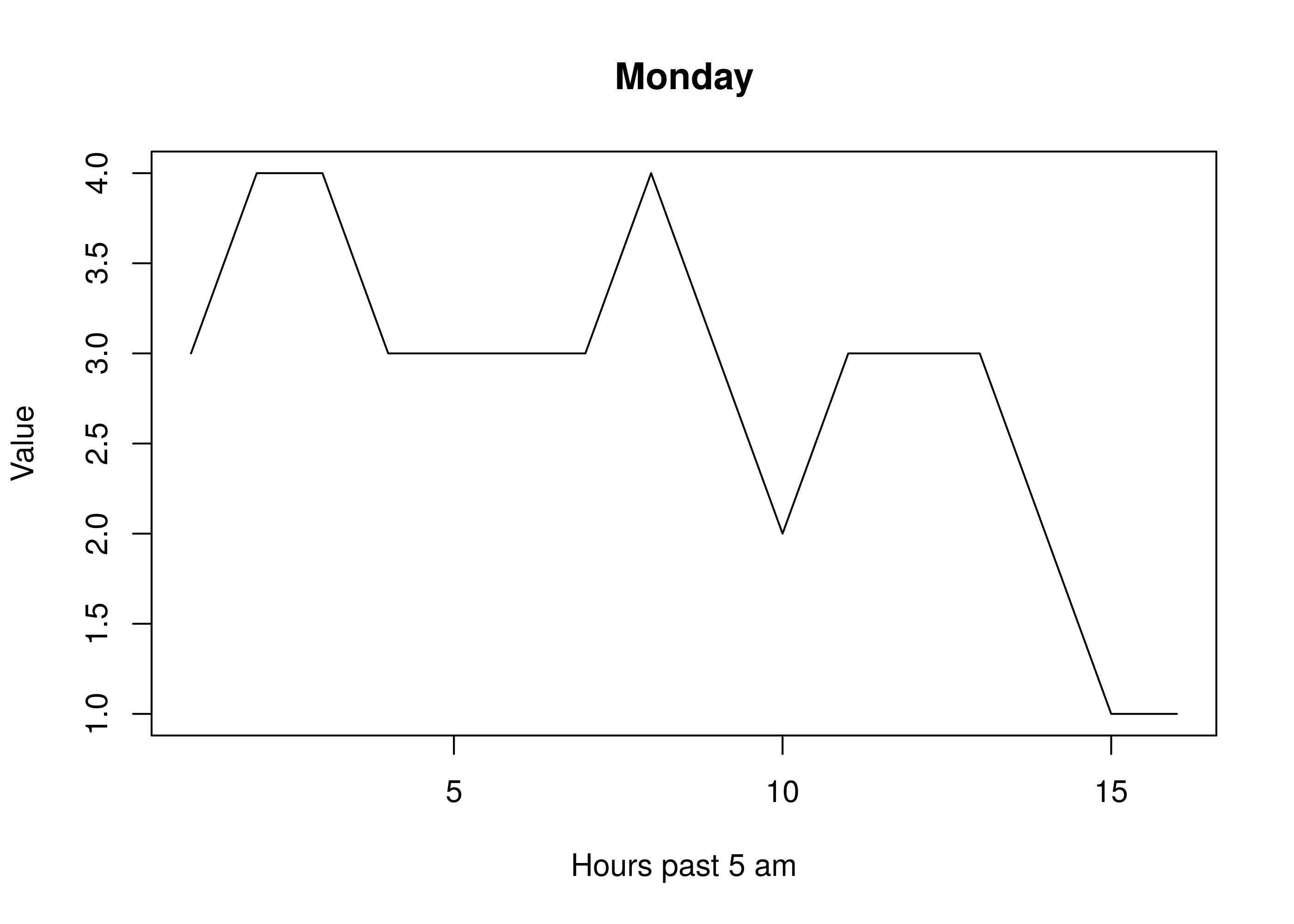Rmarkdown source

# Introduction

Among productivity bloggers there is a popular idea that the existence of so-called “ultradian rhythms” implies that one can usefully schedule one’s work time around such rhythms (see eg. , ,  and ). The only plausible citation from the scientific literature that I have found in these blogs is Ericsson, 1993, which indicates that high-performing violinsts and students of the violin rated as “good” may tend to practice (in the aggrigate) more in the morning, and then later in the afternoon [5, pg. 376], creating a time graph with two peaks.

In order to determine the best times of the day to try to be productive (as suggested in broad outline in the Asian Efficiency blog  I rated my subjective sense of productivity every hour on the hour for seven days, from Saturday morning at 5 am through the following Friday at 8 pm. I logged every hour from 5 am to 8 pm each week day, and from 10 am to 8 pm on the weekend days, this roughly coresponding to my hours of wakefulness.

The scale used was a qualitative scale, notionally from 0 to 5, although zero, corresponding to being dead, asleep or in a coma, was never used, and 5, corresponding to something like psychotic mania was also not used, so the scale effectively ran from 1 to 4, with 4 denoting great productivity and 1 denoting very little. The question asked in collecting the data was “how productive do I feel like I am being or could be if allowed the opportunity.” The question was not just “how productive would I like to be?” as the point was to measure actual physiological or psychical arrousal. The question was also not, “how productive am I being right now?” as it was not always possible to be working even if I wanted to be. Also note that questions such as “how to I feel?” and “am I really getting something valuable done” are not at issue. The Asian Efficiency blog referenced above recommends recording three separate variables: “Each hour, write down in the box your level of physical energy, concentration, and mood.” My approach wraps all three into the single variable, as the issue of interest is simply, “Am I feeling willing and able to be productive right now?”

Let `data` be the table of data. Column 1 (`day.of.the.week`) is a numeric code denoting the day of the week (Monday = 1); column 2 (`hour`) is the hour number in 24-hour format; column 3 (`status`) is the dependent variable of interest; column 4 is a text string with notes. We split the data into seven individual days.

Raw data is in the file status-log.csv in the same directory as this file.

``````setwd("./")
mo <- data[data\$day.of.the.week == 1,]
tu <- data[data\$day.of.the.week == 2,]
we <- data[data\$day.of.the.week == 3,]
th <- data[data\$day.of.the.week == 4,]
fr <- data[data\$day.of.the.week == 5,]
sa <- data[data\$day.of.the.week == 6,]
su <- data[data\$day.of.the.week == 7,]
sd <- sd(data\$status)
m <- mean(data\$status)``````

The data consists of 102 observations. If `status` were symetrically distrubuted, we would expect the mean to be around 2.5. The observed mean is 2.71 (SD = 0.9), well within 1 SD of expected.

# Analysis

First, we generate a basic line plot for all the data. It’s value is only in showing that there is plausibly a basic periodicity to the data:

``plot(data\$status, type="l", main="All data", ylab="Value", xlab="Observation Number")``Next, we break the data into a plot for each of the seven days:

``````ylabel <- "Value"
xlab_weekdays <- "Hours past 5 am"
xlab_weekends <- "Hours past 10 am"
plot(mo\$status, type="l", main = "Monday", xlab=xlab_weekdays, ylab=ylabel)````````plot(tu\$status, type="l", main = "Tuesday", xlab=xlab_weekdays, ylab=ylabel)``